# How to Convert Categorical Variables Into Dummy Variables

Knoji reviews products and up-and-coming brands we think you'll love. In certain cases, we may receive a commission from brands mentioned in our guides. Learn more.
How to change nominal or categorical variables into dummy variables to facilitate statistical analysis of data by the computer.

Students of statistics are usually confronted with issues on how to convert nominal or categorical variables into dummy variables. This is a required step to do before data is encoded into the computer so that the computer is able to 'understand' and analyze a given set of data using statistical softwares. Statistical analysis is employed to determine if there are relationships or differences between samples obtained during the course of a research work.

What is a variable?

A variable is a quantity that can assume any of a set of values. This is a term used in research and statistics in order to simplify an otherwise complex phenomena observed in nature. A variable should be measurable, that is, it must be in terms of numbers that will then be subjected to statistical analysis.

Age, for example, can be easily encoded into the computer because age assumes a number to represent how long someone or something has existed. The same is true with height. Height can be measured in terms of meters or feet, also in numbers. But how about those variables in categories like gender? Gender is composed of males and females. But these are not in numbers. Variables like this are called nominal or categorical variables.

There is therefore a need for a nominal or categorical variable like gender to be converted into something that the computer can understand. Computers basically work in binary mode. This means that computers 'think' in base two. Ones and zeros; on and off. Therefore, data must be converted into the binary form.

Dummy Variables

At this point, dummy variables are necessary to allow analysis of nominal or categorical variables like gender. The two categories of gender, that is, male and female can be represented by the numbers "1" and "0". The male category may be represented by the number "1" while the female category by the number "0". This means that if you encode "1" into a spreadsheet this means male is represented, not the female. When a female is represented, "0" must be entered. Gender is thus represented as the dummy variable 'X1" in the matrix below.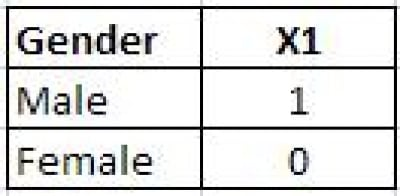But how about if the categories are more than two? Say for example, eye color? How can this be represented as dummy variables?

The principle is still the same but much more easily understood using again a matrix. A set of dummy variables are generated below to represent eye color. These dummy variables are 'X1' and 'X2' to represent eye color.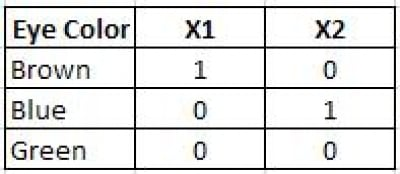It is now then possible to analyze the data given the set of numbers that represent the nominal or categorical variables. Nominal or categorical variables are converted into dummy variables in binary form that facilitate statistical analysis by computers.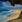chy99
0
Posted on Jul 20, 2010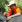Patrick Regoniel
1
Posted on Jul 19, 2010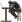deepblue
0
Posted on Jul 19, 2010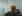Ron Siojo
0
Posted on Jul 18, 2010Patrick Regoniel
1
Posted on Jul 17, 2010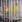Phoenix Montoya
0
Posted on Jul 17, 2010